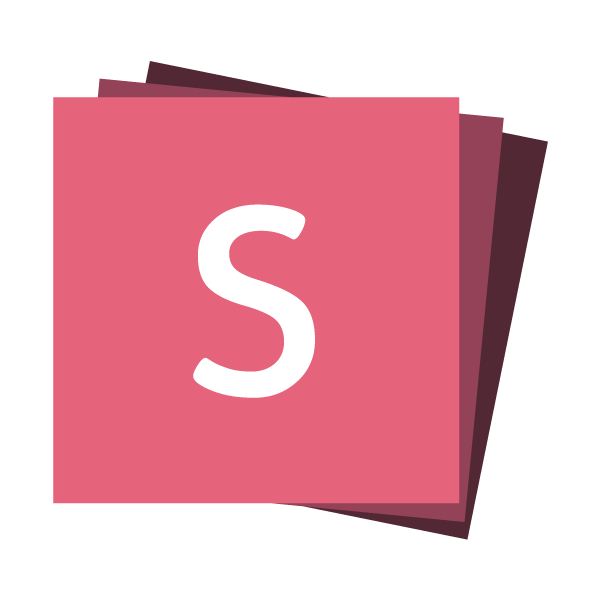## Fit Text

Resizes text to be as large as possible within its container.

``````
FIT-TEXT
``````

## Stretch

Makes an element as tall as possible while remaining within the slide bounds.

``````
Stretch ExampleImage byline
``````

## Stretch ExampleImage byline

## Stack

Stacks multiple elements on top of each other, for use with fragments.

``````

<img class="fragment" width="450" height="300" src="...">
<img class="fragment" width="300" height="450" src="...">
<img class="fragment" width="400" height="400" src="...">

``````

## Stack Example## Stack Example

One at a time.## HStack

Stacks multiple elements horizontally.

``````

<img width="450" height="300" src="...">
<img width="300" height="450" src="...">
<img width="400" height="400" src="...">

``````

One

Two

Three